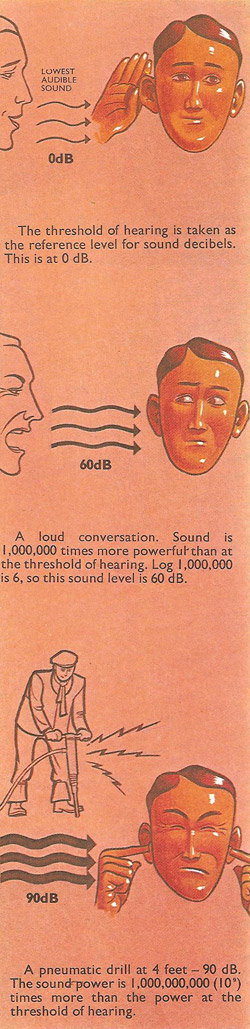A# decibel (dB)A decibel (dB) is a logarithmic unit (see logarithm) used to express power ratio, equal to one-tenth of a bel, widely used in the fields of acoustics and telecommunications. A power source of intensity P has a power level of 10log10P/P0 decibels, where P0 is the intensity of a reference source.

The decibel is commonly employed in describing noise levels relative to the threshold of hearing. Doubling the noise level adds 30 to the decibel rating. The faintest audible sound (corresponding to an excess air pressure of 2 × 10-5 pascal) is given an arbitrary value of 0 dB; a whisper has an intensity of about 30 dB, normal speech 50–60 dB, a shout 90 dB, and a jet aircraft 120 dB (about the same as the human pain threshold).

## Understanding the bel and decibel

These units are difficult to understand for two reasons. First, they are not units like the centimeter or gram, which have a definite, fixed value. They are a measure of an output power compared with some other power level used as a reference. There is a great deal of confusion over the reference levels, and there are no generally accepted standards. The second difficulty is that bel and decibel are logarithmic units. When the output power is ten times bigger than the reference power, it is expressed as one bel (or 10 decibels). 1 is the logarithm (to base 10) of 10.

However, if the output power is a hundred times bigger, it becomes only 2 bels, or 20 decibels, The logarithm of 100 is 2. In the same way,a power amplification of 3 bels (30 decibels) means a thousandfold increase in power. The logarithm of 1,000 is 3 (the number of noughts after the first figure).

The human ear is capable of hearing notes over an extensive range of loudness. One common reference level for the decibel scale is taken as the softest sound power level that the ear can detect. The loudest sound that the ear can stand is then about 13 bels. This may not sound very much, but it in fact means that the power associated with the sound is 10 trillion times the power at the soft end of the range.

Decibels can be used to compare two currents, two voltages, two powers, two intensities of sound, or two sound pressures. In fact they are a measure of the gain in any physical system.

The gain of an amplifier is sometimes quoted in decibels. Many pieces of audio equipment contain several amplifiers. The total gain is the result of multiplying together the individual gains. When these are quoted as logarithms, it is an easy matter to find the total gain by adding the logarithms. Adding logarithms has the same effect as multiplying ordinary numbers.